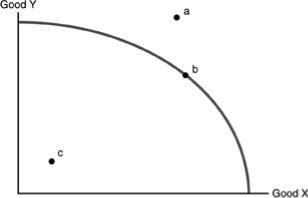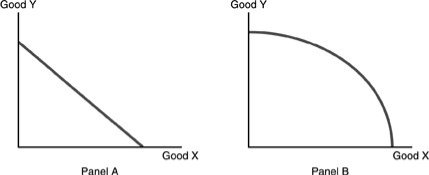• FAQ
• Contact/ Homework Answers / Economics / 61) Whenever the economy producing the maximum amount of goods
Not my Question
Flag Content

Question

61) Whenever the economy is producing the maximum amount of goods given the level of technology and the amount of resources

A) the number of resources used should be decreased since society must protect its scarce resources.

B) the concept of opportunity costs does not exist.

C) more goods will be available than customers want to buy.

D) the economy is producing efficiently.62) Refer to the above figure. Assume that only two goods can be produced in the economy. Which of the following statements is TRUE?

A) Points a, b, and c are all obtainable points of production.

B) Points a and b are obtainable points of production.

C) Points b and c are obtainable points of production.

D) Only point b is an obtainable and efficient point of production.

63) Refer to the above figure. Given our current level of technology and resources, the unobtainable point(s) of production would be

A) points a and c.

B) point a.

C) point b.

D) point c.

64) Refer to the above figure. We are currently producing at point c. Which of the following statements is TRUE?

A) Resources are not being efficiently utilized.

B) Resources are being efficiently utilized.

C) The only way to produce more of Goods X or Y is to have an increase in the amount of resources.

D) The Law of Increasing Additional Cost does not hold.

65) All mutually beneficial trades have taken place. This implies that

A) the production possibilities curve is bowed out.

B) society is inside the production possibilities curve.

C) economic efficiency prevails in the society.

D) society is on the constant cost portion of its production possibilities curve.

66) Technology is

A) society's pool of knowledge of how to produce goods and services.

B) a resource like land or physical capital.

C) computers and lasers.

D) not obtainable by engaging in activities that increase human capital.

67) The law of increasing additional cost is due to

A) scarcity.

B) inefficient use of technology.

C) the fact that resources are not perfectly adaptable for alternative uses.

D) the fact that there are always alternatives and it is costly to figure out which alternative is best.

68) The reason the production possibilities curve is bowed outward (concave) is

A) the law of increasing additional cost.

B) that technology is constantly changing.

C) that the number of resources is increasing.

D) that the economy has more capital goods than entrepreneurial effort.

69) Some resources are better suited to produce certain goods than other resources. This fact leads to

A) a linear production possibilities curve.

B) inefficiency in the economy.

C) the law of increasing additional cost.

D) increases in technology.70) Refer to the above figure. The Law of Increasing Additional Costs applies in

A) Panel A only.

B) Panel B only.

C) Panels A and B.

D) neither Panel A nor B.

Solution 5 (1 Ratings )

Solved
Economics 1 Month Ago 2 Views
This Question has Been Answered!